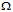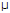# Digital Electronics - Digital Signal Processing

### Exercise :: Digital Signal Processing - General Questions

11.

Which type of ADC quantizes the analog signal into a stream of bits whose amount corresponds to the signal level?

 A. Successive approximation B. Sigma-delta C. Dual-slope D. None of the above

Answer: Option B

Explanation:

No answer description available for this question. Let us discuss.

12.

A binary-weighted-input digital-to-analog converter has an input resistor of 100 k. If the resistor is connected to a 5 V source, current through the resistor is ________.

 A. 50 mA B. 50A C. 5 mA D. 500A

Answer: Option B

Explanation:

No answer description available for this question. Let us discuss.

13.

In troubleshooting a DAC, we check its performance characteristics, such as ________.

 A. nonmonotonicity B. differential nonlinearity C. low and high gain D. all of the above

Answer: Option D

Explanation:

No answer description available for this question. Let us discuss.

14.

In a digital reproduction of an analog curve, accuracy can be increased by ________.

 A. sampling the curve more often B. sampling the curve less often C. decreasing the number of bits used to represent each sampled value D. all of the above

Answer: Option A

Explanation:

No answer description available for this question. Let us discuss.

15.

In a 4-bit R/2R ladder digital-to-analog converter, because of negative feedback, the operational amplifier keeps the inverting (minus) input near ________.

 A. 5 volts B. zero volts C. a voltage determined by the binary weighted input D. none of the above

Answer: Option B

Explanation:

No answer description available for this question. Let us discuss.

#### Current Affairs 2021

Interview Questions and Answers